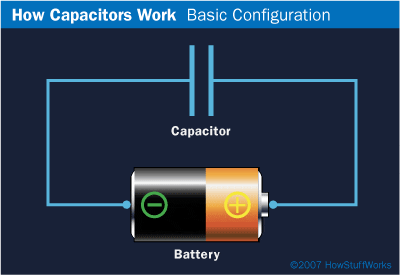# Conductors in capacitor equal but opposite charge?

• helios1234
In summary, the reason for the equal and opposite charge on the conductors of a capacitor is due to the constant electric field across the capacitor. If the charges were not equal, it would create a local electric field and disrupt the flow of electrons. This principle also applies to the flow of electrons in a battery. By understanding the concept of electric fields, one can better understand the flow of electrons in a circuit and in different components.f

## Homework Statement

If you put a capacitor in some circuit, why exactly do the conductors that form the capacitor have equal and opposite charge? This seems to be assumed in many cases.

## Homework Equations

Is it possible for the conductors to have different magnitude of charge?

You can't accumulate relevant amounts of charges anywhere in a circuit. Any accumulated charge would repel additional charge quickly. In practice you can assume all components stay without a net charge (unless you go to extremely low current or high frequency applications).

Hey @helios1234 , for every electron that enters the capacitor plate connected to the negative terminal of the battery, one electron leaves the positive side of the battery, so they end up having equal charge.

As for why that happens: you've probably heard an electric field is constant across a capacitor, so it would actually be strange if it didn't happen since on either side electrons will have the same "push" or "pull" being provided by the battery.

You may as well ask why it is that electrons in a battery enter the positive side at roughly the same rate they leave the negative side. Imagine if they didn't. Local bunching up of electrons would create electric fields that in different parts of the wire where electrons were entering at a faster rate than they were leaving, therefore speeding up electrons in that area until they were all moving at around the same average speed again.

The same applies to capacitor plate: if electrons bunched up, even for a tiny bit, on a single plate, it would create a local electric field and therefore slow down the rate of electrons entering the negative plate for a millisecond and speed up those leaving the positive plate for a millisecond until the charge on each was the same (remember, the magnitude of an electric field is proportional to the amount of charge in some area, and you may not know this yet but the magnitude of the electric field is proportional to the drift velocity of the electrons, which is basically on average how fast they move from one end of the circuit to the other. I think is always wise to read more about electric fields in a circuit and in different components in general, because that truly helps you understand the flow of electrons better).

I hope that made sense, tell me if you need some clarification.

Cheers!
-Josh#### Attachments

The same current flows from the - plate to the + plate so you have to have the same number of charges on each plate.